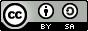# 机器翻译¶

## 说明¶

1. 硬件要求 本文可支持在CPU、GPU下运行
2. 对docker file cuda/cudnn的支持 如果您使用了本文配套的docker镜像，请注意：该镜像对GPU的支持仅限于CUDA 8，cuDNN 5
3. 文档中代码和seq2seq.py不一致的问题 请注意：为使本文更加易读易用，我们拆分、调整了seq2seq.py的代码并放入本文。本文中代码与seq2seq.py的运行结果一致，如希望直接看到训练脚本输出效果，可运行seq2seq.py

## 背景介绍¶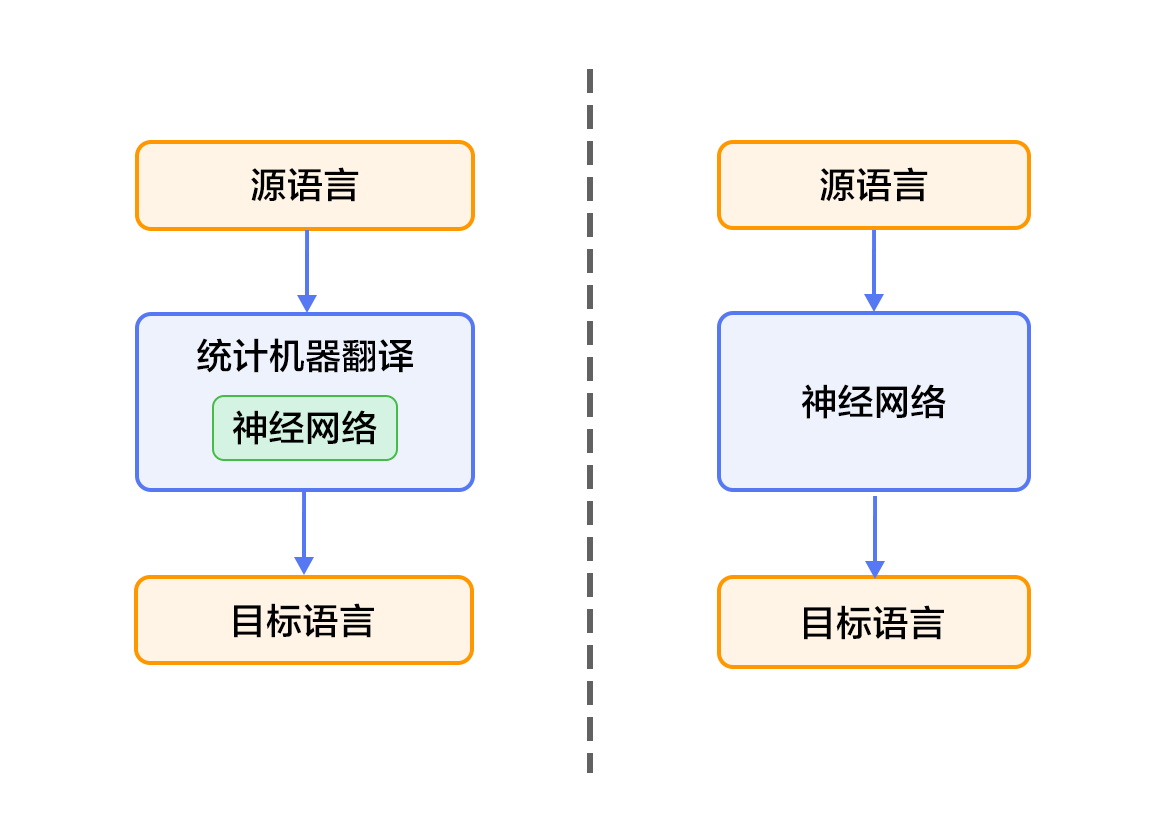## 效果展示¶

这些 是 希望 的 曙光 和 解脱 的 迹象 .


0 -5.36816   These are signs of hope and relief . <e>
1 -6.23177   These are the light of hope and relief . <e>
2 -7.7914  These are the light of hope and the relief of hope . <e>

• 左起第一列是生成句子的序号；左起第二列是该条句子的得分（从大到小），分值越高越好；左起第三列是生成的英语句子。
• 另外有两个特殊标志：<e>表示句子的结尾，<unk>表示未登录词（unknown word），即未在训练字典中出现的词。

## 模型概览¶

### GRU¶

GRU是Cho等人在LSTM上提出的简化版本，也是RNN的一种扩展，如下图所示。GRU单元只有两个门：

• 重置门（reset gate）：如果重置门关闭，会忽略掉历史信息，即历史不相干的信息不会影响未来的输出。
• 更新门（update gate）：将LSTM的输入门和遗忘门合并，用于控制历史信息对当前时刻隐层输出的影响。如果更新门接近1，会把历史信息传递下去。### 双向循环神经网络¶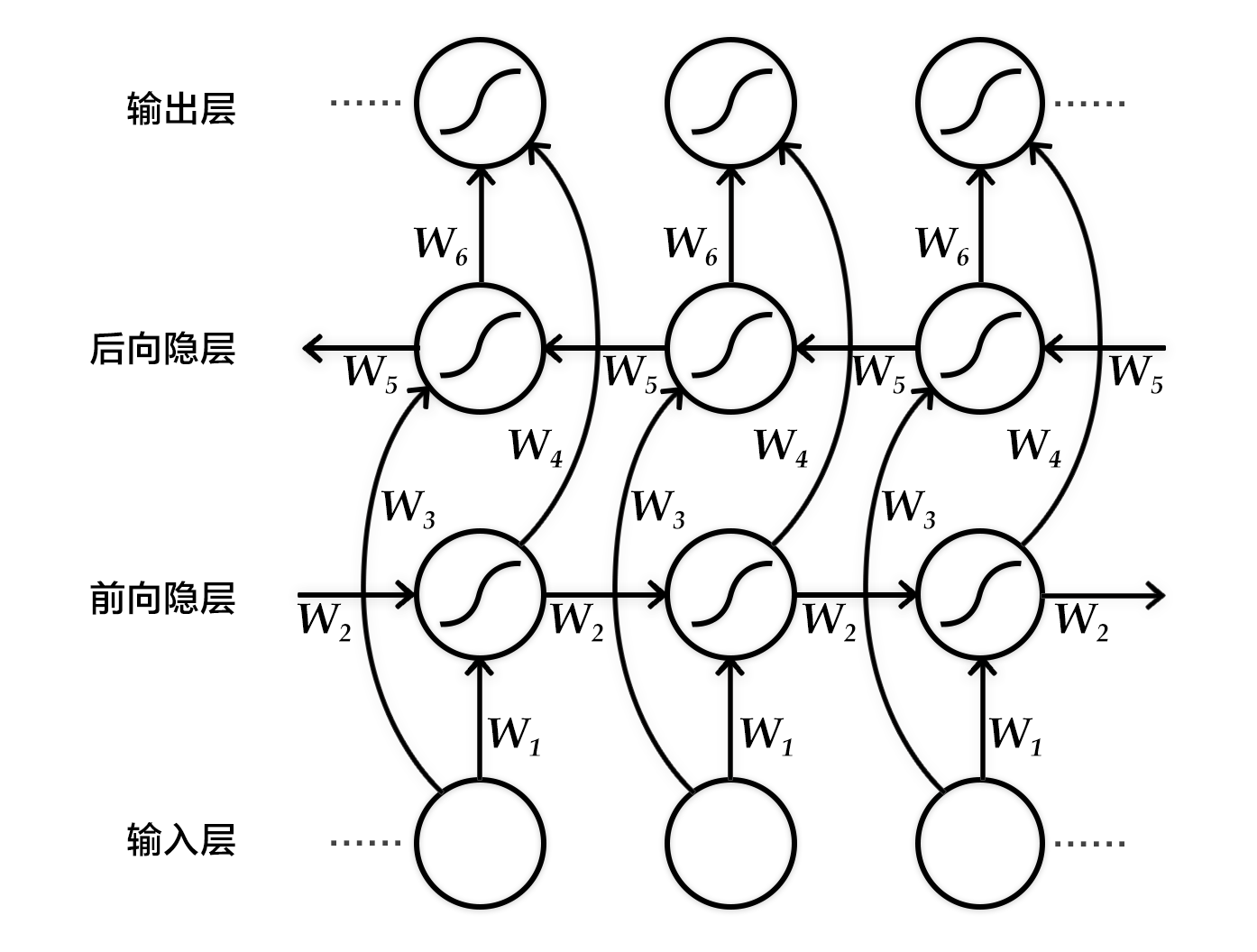### 编码器-解码器框架¶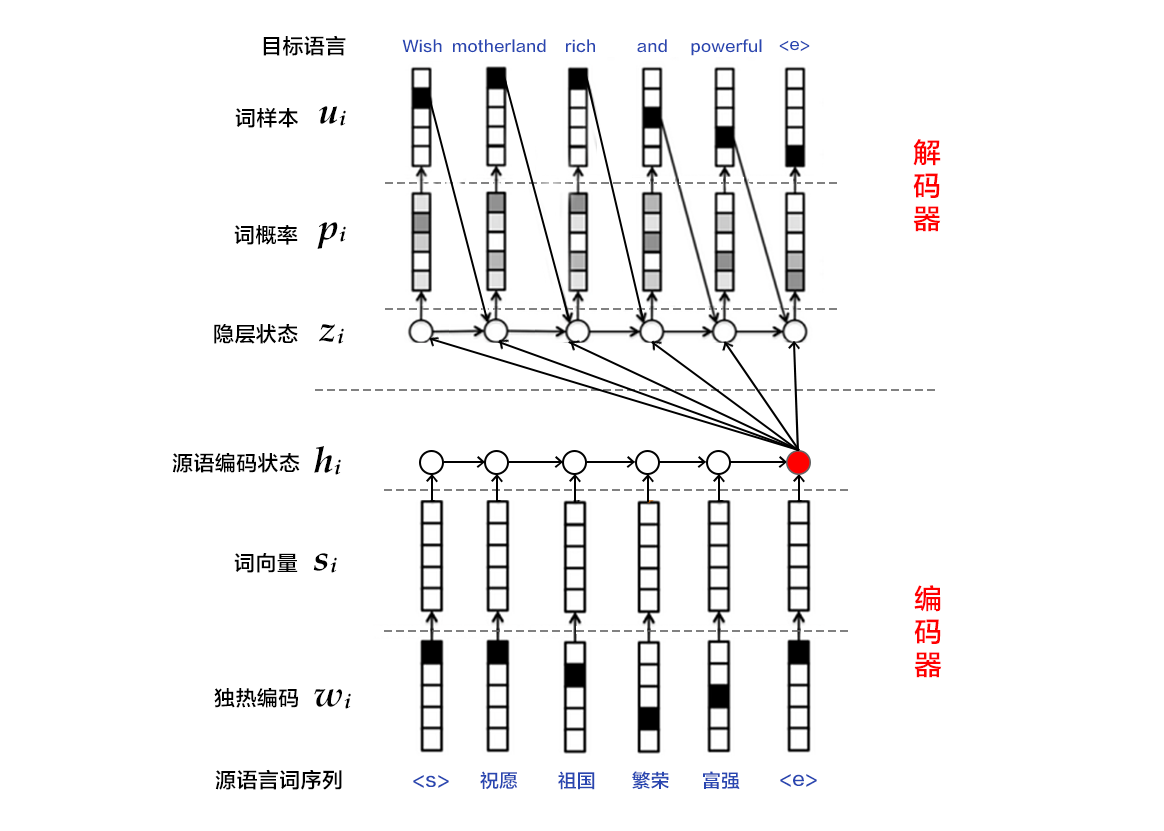#### 编码器¶

1. one-hot vector表示：将源语言句子$x=\left { x_1,x_2,...,x_T \right }$的每个词$x_i$表示成一个列向量$w_i\epsilon \left { 0,1 \right }^{\left | V \right |},i=1,2,...,T$。这个向量$w_i$的维度与词汇表大小$\left | V \right |$ 相同，并且只有一个维度上有值1（该位置对应该词在词汇表中的位置），其余全是0。
2. 映射到低维语义空间的词向量：one-hot vector表示存在两个问题，1）生成的向量维度往往很大，容易造成维数灾难；2）难以刻画词与词之间的关系（如语义相似性，也就是无法很好地表达语义）。因此，需再one-hot vector映射到低维的语义空间，由一个固定维度的稠密向量（称为词向量）表示。记映射矩阵为$C\epsilon R^{K\times \left | V \right |}$，用$s_i=Cw_i$表示第$i$个词的词向量，$K$为向量维度。
3. 用RNN编码源语言词序列：这一过程的计算公式为$h_i=\varnothing \theta \left ( h_{i-1}, s_i \right )$，其中$h_0$是一个全零的向量，$\varnothing _\theta$是一个非线性激活函数，最后得到的$\mathbf{h}=\left { h_1,..., h_T \right }$就是RNN依次读入源语言$T$个词的状态编码序列。整句话的向量表示可以采用$\mathbf{h}$在最后一个时间步$T$的状态编码，或使用时间维上的池化（pooling）结果。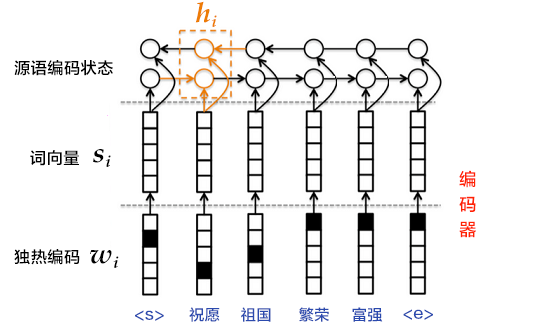#### 解码器¶

1. 每一个时刻，根据源语言句子的编码信息（又叫上下文向量，context vector）$c$、真实目标语言序列的第$i$个词$u_i$和$i$时刻RNN的隐层状态$z_i$，计算出下一个隐层状态$z_{i+1}$。计算公式如下：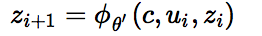1. 将$z_{i+1}$通过softmax归一化，得到目标语言序列的第$i+1$个单词的概率分布$p_{i+1}$。概率分布公式如下：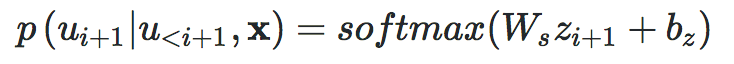1. 根据$p_{i+1}$和$u_{i+1}$计算代价。
2. 重复步骤1~3，直到目标语言序列中的所有词处理完毕。

### 注意力机制¶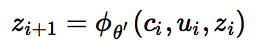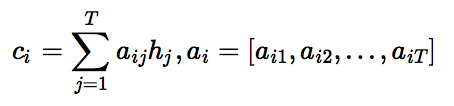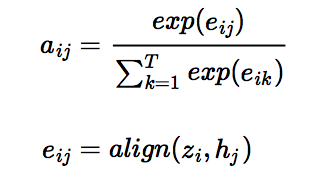### 柱搜索算法¶

1. 每一个时刻，根据源语言句子的编码信息$c$、生成的第$i$个目标语言序列单词$u_i$和$i$时刻RNN的隐层状态$z_i$，计算出下一个隐层状态$z_{i+1}$。
2. 将$z_{i+1}$通过softmax归一化，得到目标语言序列的第$i+1$个单词的概率分布$p_{i+1}$。
3. 根据$p_{i+1}$采样出单词$u_{i+1}$。
4. 重复步骤1~3，直到获得句子结束标记<e>或超过句子的最大生成长度为止。

## 数据介绍¶

### 数据预处理¶

• 将每个源语言到目标语言的平行语料库文件合并为一个文件：
• 合并每个XXX.srcXXX.trg文件为XXX
• XXX中的第$i$行内容为XXX.src中的第$i$行和XXX.trg中的第$i$行连接，用'\t'分隔。
• 创建训练数据的“源字典”和“目标字典”。每个字典都有DICTSIZE个单词，包括：语料中词频最高的（DICTSIZE - 3）个单词，和3个特殊符号<s>（序列的开始）、<e>（序列的结束）和<unk>（未登录词）。

## 模型配置说明¶

from __future__ import print_function
import os
import six

import numpy as np

dict_size = 30000  # 词典大小
bos_id = 0  # 词典中start token对应的id
eos_id = 1  # 词典中end token对应的id
source_dict_size = target_dict_size = dict_size  # 源/目标语言字典大小
word_dim = 512  # 词向量维度
hidden_dim = 512  # 编码器中的隐层大小
decoder_size = hidden_dim  # 解码器中的隐层大小
max_length = 256  # 解码生成句子的最大长度
beam_size = 4  # beam search的柱宽度
batch_size = 64  # batch 中的样本数

model_save_dir = "machine_translation.inference.model"


def data_func(is_train=True):
# 源语言source数据
src = fluid.data(name="src", shape=[None, None], dtype="int64")
src_sequence_length = fluid.data(name="src_sequence_length",
shape=[None],
dtype="int64")
inputs = [src, src_sequence_length]
# 训练时还需要目标语言target和label数据
if is_train:
trg = fluid.data(name="trg", shape=[None, None], dtype="int64")
trg_sequence_length = fluid.data(name="trg_sequence_length",
shape=[None],
dtype="int64")
label = fluid.data(name="label", shape=[None, None], dtype="int64")
inputs += [trg, trg_sequence_length, label]
capacity=10,
iterable=True,
use_double_buffer=True)


def encoder(src_embedding, src_sequence_length):
# 使用GRUCell构建前向RNN
encoder_fwd_cell = layers.GRUCell(hidden_size=hidden_dim)
encoder_fwd_output, fwd_state = layers.rnn(
cell=encoder_fwd_cell,
inputs=src_embedding,
sequence_length=src_sequence_length,
time_major=False,
is_reverse=False)
# 使用GRUCell构建反向RNN
encoder_bwd_cell = layers.GRUCell(hidden_size=hidden_dim)
encoder_bwd_output, bwd_state = layers.rnn(
cell=encoder_bwd_cell,
inputs=src_embedding,
sequence_length=src_sequence_length,
time_major=False,
is_reverse=True)
# 拼接前向与反向GRU的编码结果得到h
encoder_output = layers.concat(
input=[encoder_fwd_output, encoder_bwd_output], axis=2)
encoder_state = layers.concat(input=[fwd_state, bwd_state], axis=1)
return encoder_output, encoder_state


• 首先通过 Cell 定义解码器中单步的计算，即$z_{i+1}=\phi _{\theta '}\left ( c_i,u_i,z_i \right )$，这里使用 GRU 并加上注意力机制（Additive Attention），代码如下：

class DecoderCell(layers.RNNCell):
def __init__(self, hidden_size):
self.hidden_size = hidden_size
self.gru_cell = layers.GRUCell(hidden_size)

def attention(self, hidden, encoder_output, encoder_output_proj,
# 定义attention用以计算context，即 c_i，这里使用Bahdanau attention机制
decoder_state_proj = layers.unsqueeze(
layers.fc(hidden, size=self.hidden_size, bias_attr=False), )
encoder_output_proj,
layers.expand(decoder_state_proj,
[1, layers.shape(decoder_state_proj), 1]))
attn_scores = layers.squeeze(
layers.fc(input=mixed_state,
size=1,
num_flatten_dims=2,
bias_attr=False), )
attn_scores = layers.softmax(attn_scores)
context = layers.reduce_sum(layers.elementwise_mul(encoder_output,
attn_scores,
axis=0),
dim=1)
return context

def call(self,
step_input,
hidden,
encoder_output,
encoder_output_proj,
# Bahdanau attention
context = self.attention(hidden, encoder_output, encoder_output_proj,
step_input = layers.concat([step_input, context], axis=1)
# GRU
output, new_hidden = self.gru_cell(step_input, hidden)
return output, new_hidden

• 基于定义的单步计算，使用 fluid.layers.rnnfluid.layers.dynamic_decode 分别实现用于训练和预测生成的多步循环解码器，如下：

def decoder(encoder_output,
encoder_output_proj,
encoder_state,
trg=None,
is_train=True):
# 定义 RNN 所需要的组件
decoder_cell = DecoderCell(hidden_size=decoder_size)
decoder_initial_states = layers.fc(encoder_state,
size=decoder_size,
act="tanh")
trg_embeder = lambda x: fluid.embedding(input=x,
size=[target_dict_size, hidden_dim],
dtype="float32",
param_attr=fluid.ParamAttr(
name="trg_emb_table"))
output_layer = lambda x: layers.fc(x,
size=target_dict_size,
num_flatten_dims=len(x.shape) - 1,
param_attr=fluid.ParamAttr(name=
"output_w"))
if is_train:  # 训练
# 训练时使用 layers.rnn 构造由 cell 指定的循环神经网络
# 循环的每一步从 inputs 中切片产生输入，并执行 cell.call
decoder_output, _ = layers.rnn(
cell=decoder_cell,
inputs=trg_embeder(trg),
initial_states=decoder_initial_states,
time_major=False,
encoder_output=encoder_output,
encoder_output_proj=encoder_output_proj,
decoder_output = output_layer(decoder_output)
else:  # 基于 beam search 的预测生成
# beam search 时需要将用到的形为 [batch_size, ...] 的张量扩展为 [batch_size* beam_size, ...]
encoder_output = layers.BeamSearchDecoder.tile_beam_merge_with_batch(
encoder_output, beam_size)
encoder_output_proj = layers.BeamSearchDecoder.tile_beam_merge_with_batch(
encoder_output_proj, beam_size)
# BeamSearchDecoder 定义了单步解码的操作：cell.call + beam_search_step
beam_search_decoder = layers.BeamSearchDecoder(cell=decoder_cell,
start_token=bos_id,
end_token=eos_id,
beam_size=beam_size,
embedding_fn=trg_embeder,
output_fn=output_layer)
# 使用 layers.dynamic_decode 动态解码
# 重复执行 decoder.step() 直到其返回的表示完成状态的张量中的值全部为True或解码步骤达到 max_step_num
decoder_output, _ = layers.dynamic_decode(
decoder=beam_search_decoder,
inits=decoder_initial_states,
max_step_num=max_length,
output_time_major=False,
encoder_output=encoder_output,
encoder_output_proj=encoder_output_proj,

return decoder_output


def model_func(inputs, is_train=True):
# 源语言输入
src = inputs
src_sequence_length = inputs
src_embeder = lambda x: fluid.embedding(
input=x,
size=[source_dict_size, hidden_dim],
dtype="float32",
param_attr=fluid.ParamAttr(name="src_emb_table"))
src_embedding = src_embeder(src)

# 编码器
encoder_output, encoder_state = encoder(src_embedding, src_sequence_length)

encoder_output_proj = layers.fc(input=encoder_output,
size=decoder_size,
num_flatten_dims=2,
bias_attr=False)
maxlen=layers.shape(src),
dtype="float32")

# 目标语言输入，训练时有、预测生成时无该输入
trg = inputs if is_train else None

# 解码器
output = decoder(encoder_output=encoder_output,
encoder_output_proj=encoder_output_proj,
encoder_state=encoder_state,
trg=trg,
is_train=is_train)
return output


def loss_func(logits, label, trg_sequence_length):
probs = layers.softmax(logits)
# 使用交叉熵损失函数
loss = layers.cross_entropy(input=probs, label=label)
maxlen=layers.shape(logits),
dtype="float32")
return avg_cost

def optimizer_func():
# 设置梯度裁剪
# 定义先增后降的学习率策略
lr_decay = fluid.layers.learning_rate_scheduler.noam_decay(hidden_dim, 1000)
learning_rate=lr_decay,
regularization=fluid.regularizer.L2DecayRegularizer(
regularization_coeff=1e-4))


## 训练模型¶

### 定义数据生成器¶

def inputs_generator(batch_size, pad_id, is_train=True):
data_generator = fluid.io.shuffle(
source_dict_size, target_dict_size)
batch_generator = fluid.io.batch(data_generator, batch_size=batch_size)

seq_lengths = np.array(list(map(len, insts)), dtype="int64")
max_len = max(seq_lengths)
[inst + [pad_id] * (max_len - len(inst)) for inst in insts],
dtype="int64")

def _generator():
for batch in batch_generator():
batch_src = [ins for ins in batch]
inputs = [src_data, src_lengths]
if is_train:  #训练时包含 target 和 label 数据
batch_trg = [ins for ins in batch]
batch_lbl = [ins for ins in batch]
inputs += [trg_data, trg_lengths, lbl_data]
yield inputs

return _generator


### 构建训练程序¶

train_prog = fluid.Program()
startup_prog = fluid.Program()
with fluid.program_guard(train_prog, startup_prog):
with fluid.unique_name.guard():
# 训练时：
# inputs = [src, src_sequence_length, trg, trg_sequence_length, label]
logits = model_func(inputs, is_train=True)
loss = loss_func(logits, inputs[-1], inputs[-2])
optimizer = optimizer_func()
optimizer.minimize(loss)


### 定义训练环境¶

# 设置训练设备
use_cuda = False
places = fluid.cuda_places() if use_cuda else fluid.cpu_places()
# 设置数据源
eos_id,
is_train=True),
places=places)
# 定义执行器，初始化参数并绑定Program
exe = fluid.Executor(places)
exe.run(startup_prog)
prog = fluid.CompiledProgram(train_prog).with_data_parallel(
loss_name=loss.name)


### 训练主循环¶

EPOCH_NUM = 2
for pass_id in six.moves.xrange(EPOCH_NUM):
batch_id = 0
loss_val = exe.run(prog, feed=data, fetch_list=[loss])
print('pass_id: %d, batch_id: %d, loss: %f' %
(pass_id, batch_id, loss_val))
batch_id += 1
# 保存模型
fluid.io.save_params(exe, model_save_dir, main_program=train_prog)


## 应用模型¶

### 构建预测程序¶

infer_prog = fluid.Program()
startup_prog = fluid.Program()
with fluid.program_guard(infer_prog, startup_prog):
with fluid.unique_name.guard():
predict_seqs = model_func(inputs, is_train=False)


### 定义预测环境¶

use_cuda = False
# 设置训练设备
places = fluid.cuda_places() if use_cuda else fluid.cpu_places()
# 设置数据源
eos_id,
is_train=False),
places=places)
# 定义执行器，加载参数并绑定Program
exe = fluid.Executor(places)
exe.run(startup_prog)
prog = fluid.CompiledProgram(infer_prog).with_data_parallel()


### 测试¶

# 获取 id 到 word 映射的词典
"en", source_dict_size, reverse=True)
"de", target_dict_size, reverse=True)
# 循环测试数据
seq_ids = exe.run(prog, feed=data, fetch_list=[predict_seqs])
for ins_idx in range(seq_ids.shape):
print("Original sentence:")
src_seqs = np.array(data["src"])
print(" ".join([
src_idx2word[idx] for idx in src_seqs[ins_idx][1:]
if idx != eos_id
]))
print("Translated sentence:")
for beam_idx in range(beam_size):
seq = [
trg_idx2word[idx] for idx in seq_ids[ins_idx, :, beam_idx]
if idx != eos_id
]
print(" ".join(seq).encode("utf8"))


Original sentence:
A man in an orange hat starring at something .
Translated score and sentence:
Ein Mann mit einem orangen Schutzhelm starrt auf etwas .
Ein Mann mit einem gelben Schutzhelm starrt auf etwas .
Ein Mann mit einem gelben Schutzhelm starrt etwas an .
Ein Mann mit einem orangen Schutzhelm starrt etwas an .


## 参考文献¶

1. Koehn P. Statistical machine translation[M]. Cambridge University Press, 2009.
2. Cho K, Van Merriënboer B, Gulcehre C, et al. Learning phrase representations using RNN encoder-decoder for statistical machine translation[C]//Proceedings of the 2014 Conference on Empirical Methods in Natural Language Processing (EMNLP), 2014: 1724-1734.
3. Chung J, Gulcehre C, Cho K H, et al. Empirical evaluation of gated recurrent neural networks on sequence modeling[J]. arXiv preprint arXiv:1412.3555, 2014.
4. Bahdanau D, Cho K, Bengio Y. Neural machine translation by jointly learning to align and translate[C]//Proceedings of ICLR 2015, 2015.
5. Papineni K, Roukos S, Ward T, et al. BLEU: a method for automatic evaluation of machine translation[C]//Proceedings of the 40th annual meeting on association for computational linguistics. Association for Computational Linguistics, 2002: 311-318.